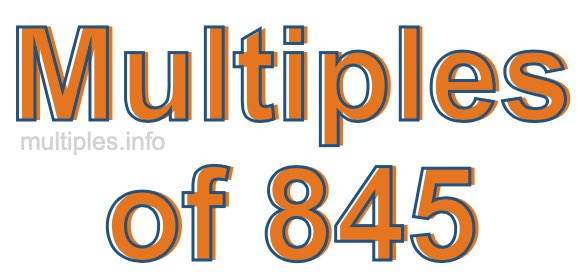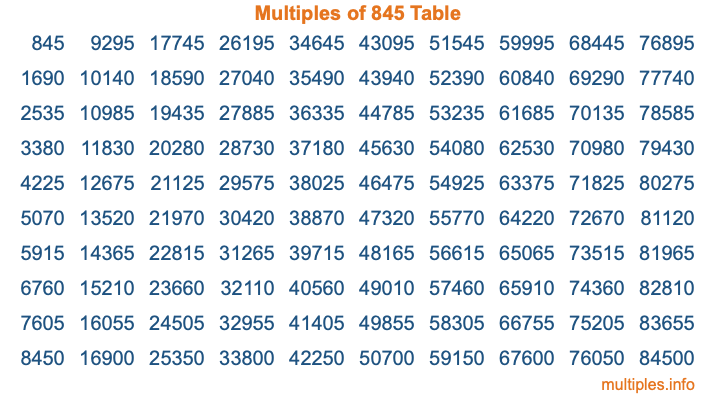Multiples of 845Welcome to the Multiples of 845 page. Here we will first teach you everything you will ever need to know about the multiples of 845, and then give you a study guide summary of everything we taught you to make sure you remember it all. Use this page to look up facts and learn information about the multiples of 845. This page will make you a multiples of eight hundred forty-five expert!

Definition of Multiples of 845
Multiples of 845 are all the numbers that when divided by 845 equal an integer. Each of the multiples of 845 are called a multiple. A multiple of 845 is created by multiplying 845 by an integer.

Therefore, to create a list of multiples of 845, you start with 1 multiplied by 845, then 2 multiplied by 845, then 3 multiplied by 845, and so on for as long as you want. Thus, the list of the first five multiples of 845 is 845, 1690, 2535, 3380, and 4225. To see a larger list of multiples of 845, see the printable image of Multiples of 845 further down on this page. We also have a category where you can choose any nth multiple of 845.

Multiples of 845 Checker
The Multiples of 845 Checker below checks to see if any number of your choice is a multiple of 845. In other words, it checks to see if there is any number (integer) that when multiplied by 845 will equal your number. To do that, we divide your number by 845. If the the quotient is an integer, then your number is a multiple of 845.

Is  a multiple of 845?

Least Common Multiple of 845 and ...
A Least Common Multiple (LCM) is the lowest multiple that two or more numbers have in common. This is also called the smallest common multiple or lowest common multiple and is useful to know when you are adding our subtracting fractions. Enter one or more numbers below (845 is already entered) to find the LCM.

Check out our LCM Calculator if you need more details about the Least Common Multiple or if you need the LCM for different numbers for adding and subtraction fractions.

nth Multiple of 845
As we stated above, 845 is the first multiple of 845, 1690 is the second multiple of 845, 2535 is the third multiple of 845, and so on. Enter a number below to find the nth multiple of 845.

th multiple of 845

Multiples of 845 vs Factors of 845
845 is a multiple of 845 and a factor of 845, but that is where the similarities end. All postive multiples of 845 are 845 or greater than 845. All positive factors of 845 are 845 or less than 845.

Below is the beginning list of multiples of 845 and the factors of 845 so you can compare:

Multiples of 845: 845, 1690, 2535, 3380, 4225, etc.

Factors of 845: 1, 5, 13, 65, 169, 845

As you can see, the multiples of 845 are all the numbers that you can divide by 845 to get a whole number. The factors of 845, on the other hand, are all the whole numbers that you can multiply by another whole number to get 845.

It's also interesting to note that if a number (x) is a factor of 845, then 845 will also be a multiple of that number (x).

Multiples of 845 vs Divisors of 845
The divisors of 845 are all the integers that 845 can be divided by evenly. Below is a list of the divisors of 845.

Divisors of 845: 1, 5, 13, 65, 169, 845

The interesting thing to note here is that if you take any multiple of 845 and divide it by a divisor of 845, you will see that the quotient is an integer.

Multiples of 845 Table
Below is an image of the first 100 multiples of 845 in a table. The table is in chronological order, column by column. The first column has the first ten multiples of 845, the second column has the next ten multiples of 845, and so on.The Multiples of 845 Table is also referred to as the 845 Times Table or Times Table of 845. You are welcome to print out our table for your studies.

Negative Multiples of 845
Although not often discussed or needed in math, it is worth mentioning that you can make a list of negative multiples of 845 by multiplying 845 by -1, then by -2, then by -3, and so on, to get the following list of negative multiples of 845:

-845, -1690, -2535, -3380, -4225, etc.

Multiples of 845 Summary
Below is a summary of important Multiples of 845 facts that we have discussed on this page. To retain the knowledge on this page, we recommend that you read through the summary and explain to yourself or a study partner why they hold true.

There are an infinite number of multiples of 845.

A multiple of 845 divided by 845 will equal a whole number.

845 divided by a factor of 845 equals a divisor of 845.

The nth multiple of 845 is n times 845.

The largest factor of 845 is equal to the first positive multiple of 845.

845 is a multiple of every factor of 845.

845 is a multiple of 845.

A multiple of 845 divided by a divisor of 845 equals an integer.

845 divided by a divisor of 845 equals a factor of 845.

Any integer times 845 will equal a multiple of 845.

Multiples of a Number
Here you can get the multiples of another number, all with the same attention to detail as we did for multiples of 845 on this page.

Multiples of
Multiples of 846
Did you find our page about multiples of eight hundred forty-five educational? Do you want more knowledge? Check out the multiples of the next number on our list!